lee上有趣题目

# 400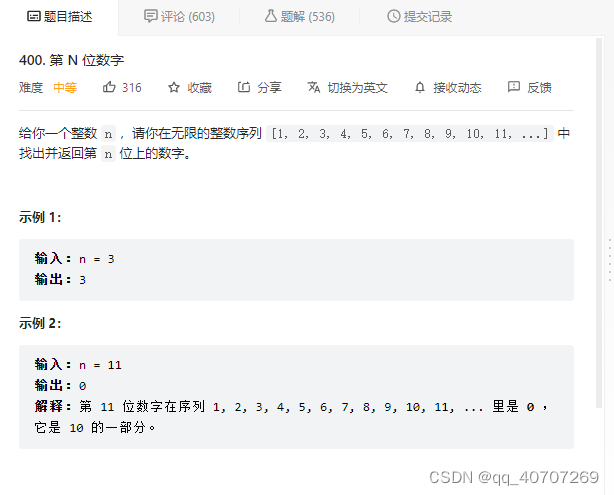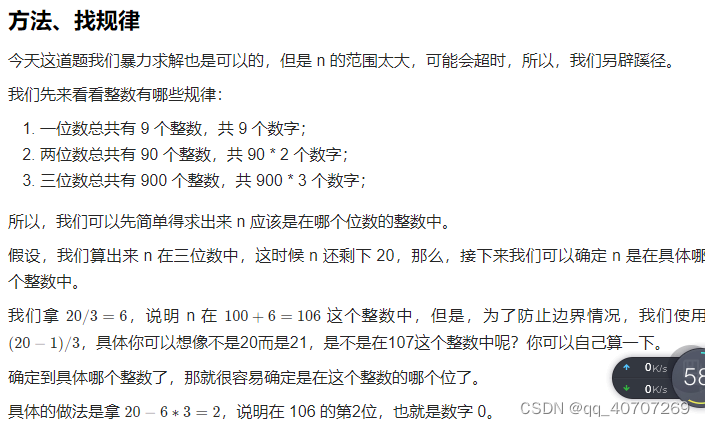`class Solution {       public int findNthDigit(int n) {           // num表示具体的整数        int num = 1;        // count表示几位数        int count = 1;        // 9 * num * count 表示几位数总共有多少位数，比如，三位数从100~999，一共是 9 * 100 * 3 = 2700 位数        while (n > 9 * (long) num * count) {               // 从小到到依次减去一位数、两位数、三位数，直到减不了为止            n -=  9 * num * count;            // num此时记录的是几位数的第一个数，依次是1、10、100、1000            num *= 10;            // count表示几位数，每经过一轮加1，表示下一次判断的位数加1            count++;        }        // 此时的 n 表示从 num 开始取第多少位        // 比如，此时n=5,num=100,count=3，表示从100开始取第5位        // 那么，100是三位，101是三位，所以，第5位肯定在101这个整数的第2位，也就是0        // num += (n - 1) / count; 用来确定是哪个具体的整数了，这里 n-1 是为了防止边界情况，比如n=3,count=3，这时候会取超了        // n -= (n - 1) / count * count; 用来确定是哪个具体的位，n=5-3=2，注意 4/3*3=3，不等于4        // count-n，上面算出来的n是从高到低的第几位，通过 count-n 转成第低到高的第几位        // (num / (int) Math.pow(10, count - n)) % 10; 取出那个位的数字                // 确定是具体哪个整数        num += (n - 1) / count;        // 确定是这个整数中的哪个位        n -= (n - 1) / count * count;        // 取出那个位的数字        return (num / (int) Math.pow(10, count - n)) % 10;    }}作者：tong-zhu链接：https://leetcode.cn/problems/nth-digit/solution/tong-ge-lai-shua-ti-la-zhao-gui-lu-by-to-nvfa/来源：力扣（LeetCode）著作权归作者所有。商业转载请联系作者获得授权，非商业转载请注明出处。`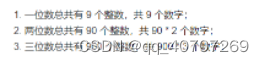`class Solution {       public int findNthDigit(int n) {           // num表示具体的整数        int num = 1;        // count表示几位数        int count = 1;        // 9 * num * count 表示几位数总共有多少位数，比如，三位数从100~999，一共是 9 * 100 * 3 = 2700 位数        while (n > 9 * (long) num * count) {               // 从小到到依次减去一位数、两位数、三位数，直到减不了为止            n -=  9 * num * count;            // num此时记录的是几位数的第一个数，依次是1、10、100、1000            num *= 10;            // count表示几位数，每经过一轮加1，表示下一次判断的位数加1            count++;        }        // 此时的 n 表示从 num 开始取第多少位        // 比如，此时n=5,num=100,count=3，表示从100开始取第5位        // 那么，100是三位，101是三位，所以，第5位肯定在101这个整数的第2位，也就是0        // num += (n - 1) / count; 用来确定是哪个具体的整数了，这里 n-1 是为了防止边界情况，比如n=3,count=3，这时候会取超了        // n -= (n - 1) / count * count; 用来确定是哪个具体的位，n=5-3=2，注意 4/3*3=3，不等于4        // count-n，上面算出来的n是从高到低的第几位，通过 count-n 转成第低到高的第几位        // (num / (int) Math.pow(10, count - n)) % 10; 取出那个位的数字                // 确定是具体哪个整数        num += (n - 1) / count;        // 确定是这个整数中的哪个位        n -= (n - 1) / count * count;        // 取出那个位的数字        return (num / (int) Math.pow(10, count - n)) % 10;    }}`

len * 9 * pow(10,len - 1)

1000 1001 1002 1003 1004 1005 1006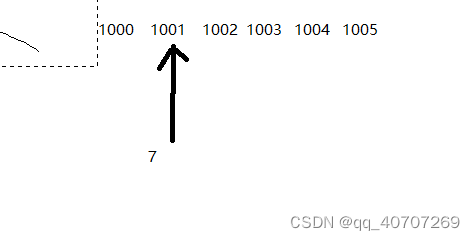7 / len 得到商是1,也就是可以定位到1000起始点往后偏移1个

ps:当len大于等于2时候，是从10或者100开始偏移的

`class Solution {       public int findNthDigit(int n) {           if(n < 10){               return n;        }        // num表示具体的整数        int num = 1;        // count表示几位数        int count = 1;        // 9 * num * count 表示几位数总共有多少位数，比如，三位数从100~999，一共是 9 * 100 * 3 = 2700 位数        while (n > 9 * (long) num * count) {               // 从小到到依次减去一位数、两位数、三位数，直到减不了为止            n -=  9 * num * count;            // num此时记录的是几位数的第一个数，依次是1、10、100、1000            num *= 10;            // count表示几位数，每经过一轮加1，表示下一次判断的位数加1            count++;        }        // 此时的 n 表示从 num 开始取第多少位        // 比如，此时n=5,num=100,count=3，表示从100开始取第5位        // 那么，100是三位，101是三位，所以，第5位肯定在101这个整数的第2位，也就是0        // num += (n - 1) / count; 用来确定是哪个具体的整数了，这里 n-1 是为了防止边界情况，比如n=3,count=3，这时候会取超了        // n -= (n - 1) / count * count; 用来确定是哪个具体的位，n=5-3=2，注意 4/3*3=3，不等于4        // count-n，上面算出来的n是从高到低的第几位，通过 count-n 转成第低到高的第几位        // (num / (int) Math.pow(10, count - n)) % 10; 取出那个位的数字                // 确定这个数位的第几个数        int pos = (n - 1) / count;        // 确定是哪个整数        //当前数位最小值 + num        int pos2 = n % count == 0 ? count : n % count;//知道是第几位        n =  (int)Math.pow(10,count - 1) + pos;//确认是谁        //                       // 取出那个位的数字        int result =  (n / (int) Math.pow(10, count - pos2)) % 10;        return result;    }}`

# 42接雨水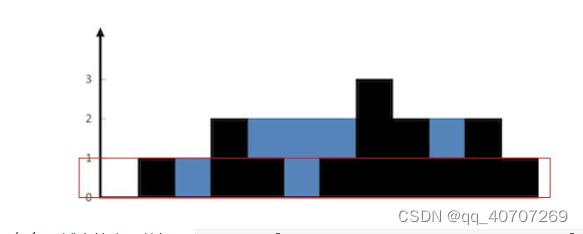`class Solution {       public int trap(int[] height) {           //需要一个flag位置为false        boolean flag = false;        int temp = 0;        int i = 1;//遍历每一层        int ans = 0;//用来返回结果        for(;;i++){               //最外层是层层增加层数            //里面一层遍历数字,            for(int value : height){                   //当当前值，小于当前层时候，就是没有墙                if(flag == false && value < i){                       continue;//直接跳过去                }                //否则flag == true，除了上面那种情况不然必然是true                flag = true;//否则flag开始计算,说明开始计算了                //如果值大于等于i就是一堵墙                //一堵墙就要将前面temp的值放进ans里，temp重新算计                if(value >= i){                       ans += temp;                    temp = 0;                                                        }                else{                       //否则就是一个数                    temp++;                }            }            //整个数组这一层遍历好，如果flag没变，说明数组没了            if(!flag){                   break;//跳出循环就得了            }            //不然重新放置回到false位置            flag = false;//            //temp也要清零，有可能有上面遗留的问题            temp = 0;        }        return ans;    }}`
`public int trap(int[] height) {       int sum = 0;    int max = getMax(height);//找到最大的高度，以便遍历。    for (int i = 1; i <= max; i++) {           boolean isStart = false; //标记是否开始更新 temp        int temp_sum = 0;        for (int j = 0; j < height.length; j++) {               if (isStart && height[j] < i) {                   temp_sum++;            }            if (height[j] >= i) {                   sum = sum + temp_sum;                temp_sum = 0;                isStart = true;            }        }    }    return sum;}private int getMax(int[] height) {   		int max = 0;		for (int i = 0; i < height.length; i++) {   			if (height[i] > max) {   				max = height[i];			}		}		return max;}作者：windliang链接：https://leetcode.cn/problems/trapping-rain-water/solution/xiang-xi-tong-su-de-si-lu-fen-xi-duo-jie-fa-by-w-8/来源：力扣（LeetCode）著作权归作者所有。商业转载请联系作者获得授权，非商业转载请注明出处。`

# 678有效括号字符串

1.当遇到 ’ ( ’ 时，’ ( ’ 所在位置的下标入栈；当遇到 ’ * ’ 时，’ * ’ 所在位置的下标入栈。

2.当遇到 ’ ) ’ 时，要令 leftStack 中的栈顶元素出栈，若此时 leftStack 为空，则继续看 starStack 是否为空，若不为空则 starStack 栈顶元素出栈，若为空返回则 false (遇到了 ’ ) '，但在这之前一个 ’ ( ’ 和 ’ * ’ 都没遇到，则一定不会匹配)。

3.当字符串全部遍历完时，若 starStack 的长度比 leftStack 的长度要小，则返回 false (有剩余的 ’ ( '，但 ’ * ’ 的数量不够了，则一定不会匹配)；否则，比较两个栈的栈顶元素值的大小，要保证 ’ ( ’ 在 ’ * ’ 的左边(starStack.peek() > leftStack.peek())才能匹配成功，当遇到满足条件的栈顶元素时，栈顶元素出栈，继续比较下一个。只要有一次该条件不满足，则直接返回 false；否则，返回 true。

`class Solution {       public boolean checkValidString(String s) {           int len = s.length();        Stack        leftStack = new Stack<>();        Stack          starStack = new Stack<>();        for (int i = 0; i < len; i++) {               // 当遇到 ' ( ' 时，' ( ' 所在位置的下标入栈            if (s.charAt(i) == '(') {                   leftStack.push(i);            // 当遇到 ' * ' 时，' * ' 所在位置的下标入栈            } else if (s.charAt(i) == '*') {                   starStack.push(i);            // 当遇到 ' ) ' 时            } else {                   // 如果leftstack不为空，先消耗'('                if (!leftStack.isEmpty()) {                       leftStack.pop();                // 如果左括号消耗完了                // '*'当左括号来用                // 检查starStack是不是空                } else if (!starStack.isEmpty()) {                       starStack.pop();                // '*'也消耗完了，现在又遇到')'，故返回false                } else {                       return false;                }             }           }        // 当字符串全部遍历完时        // 若 starStack 的长度比 leftStack 的长度要小        // 有剩余的 ' ( '，但 ' * ' 的数量不够了，则一定不会匹配        if (starStack.size() < leftStack.size()) {               return false;        // 如果剩余的 * 的数量大于等于 左括号的数量        // * 号要么当右括号来用， 要么为空         } else {               while (!starStack.isEmpty() && !leftStack.isEmpty()) {                   // 为了消耗剩下的左括号，* 的index 必须要比左括号大，才能在左括号的右边                if (leftStack.peek() > starStack.peek()) {                       return false;                }                // 消耗一对左括号和*                leftStack.pop();                starStack.pop();            }        }        return true;    }}       `

#### 最新留言

[***.219.124.196]2023年09月20日 19时01分53秒

## 关于作者喝酒易醉，品茶养心，人生如梦，品茶悟道，何以解忧？唯有杜康！
-- 愿君每日到此一游！